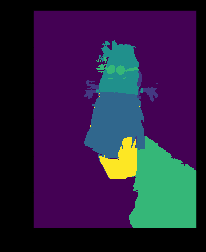# Tiramisu from great Fashion segmentation tool

Hi,

I am creating a dataset with the great tool segmentation ground truth created by Kota https://github.com/kyamagu/js-segment-annotator and would like to convert the .png file to a readable .png like in the camvid dataset.
could any one help me on this as I am stuck with python on this. Below is a simple code to go through the folder and get the encoded .png and decode them.

import numpy as np
from PIL import Image
import matplotlib
import matplotlib.pyplot as plt
import glob

images=glob.glob("*.png")

# Decode

encoded = np.array(Image.open(images))
annotation = np.bitwise_or(np.bitwise_or(
encoded[:, :, 0].astype(np.uint32),
encoded[:, :, 1].astype(np.uint32) << 8),
encoded[:, :, 2].astype(np.uint32) << 16)

print(np.unique(annotation))
plt.imshow(annotation)

output:
[0 1 2 3 4 6]

<matplotlib.image.AxesImage at 0xb0f23c8>now, the issue is getting decoded image (annotation) in a visible .png file in order to use in the tiramisu notebook.

Thank you for any help on this.

Hugues

Wow, the tool you showed is amazing. Thanks for finding it.